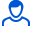Content: text (30 symbols)

Positive responses: 0
Negative responses: 0

Sold: 0
Refunds: 0Loyalty discount! If the total amount of your purchases from the seller more than:

 \$50 the discount is 10% \$100 the discount is 20% \$200 the discount is 50%

Mathematics - the science, "which studies the spatial forms and quantitative relations of the real world" (Engels).

Mathematics originated in ancient times. For the first period ("Elementary Mathematics") is characterized by:

a) immobility of these objects (length, area, volume, etc.);

b) the absence of the idea of \u200b\u200binfinity;

c) the lack of common methods.

The application areas of mathematics have been through, trade, land surveying, astronomy and architecture.

In XVII-XVIII centuries. the rapid development of production technology and the natural sciences (navigation, ballistics, hydraulics, etc.) demanded the creation of the mathematical apparatus suitable for the study variables. There was a so-called "Higher Mathematics" with its sections: analytical geometry, differential and integral calculus, differential equations, and others.

In the XIX-XX centuries. in mathematics develop new disciplines: information theory, optimal control theory, mathematical programming. The desire to simplify and speed led to the creation by the computer.

Mathematics is an exact science abstract studying quantitative relations and spatial forms. Accuracy of mathematics means that the main method in mathematical investigations are rigorous logical reasoning. Mathematical methods of research have always played and continue to play a huge role. In recent years, thanks to the emergence of high-speed computers, the use of mathematical methods has been a qualitative leap. The ability to think logically, possession of mathematical tools, the correct use of mathematics to give the arms economist or sociologist, a powerful method of investigation. This lecture course is designed for students of humanities, which will apply mathematics to solving specific economic, sociological and social problems, so drew attention to the development in the student's point of view and psychology specialists using mathematical methods in applied problems.
No feedback yet